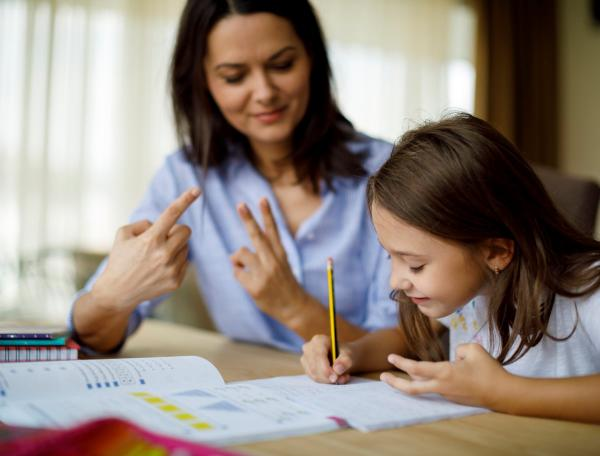# Problem? No problem!

10 - 11 yearsDeveloped in partnership with Education Services Australia

The Australian Curriculum sets the goal for what all students should learn as they progress through their school life. Skills in the Year 5-6 curriculum include:

• solving problems and checking calculations using efficient mental and written strategies
• selecting and applying efficient mental, written and calculator strategies to solve addition and subtraction word problems, including numbers with more than four digits
• using mental and written strategies to multiply up to four-digit numbers by one-digit numbers (eg 3622 x 8), then four-digit numbers by two-digit numbers (eg 3622 x 84)
• using mental and written strategies to divide a number with three or more digits by a one-digit divisor (eg 34 674 ÷ 6)
• using estimation and rounding to check the reasonableness of answers to calculations, particularly when using digital technologies such as calculators.

It’s easy to help your child practise these skills as part of everyday life – just use these simple ideas.

One of the secrets to being confident with maths is having a toolkit of different calculation strategies, and knowing when and how to use them. Involving your child in the maths problems you come across in daily life helps them develop their own maths toolkit. For example:

Could you work out how much edging we need to buy to go around the new garden bed? It’s going to be three metres long and 1.5 metres wide.
Petrol today costs \$1.65 per litre and we needed 56 litres, so how much will that be, roughly?
• show how you choose different strategies by talking through maths problems as you work them out
There are ten kids coming to your party, plus us makes fourteen people altogether. This recipe makes six muffins, double that is twelve muffins, which is not enough. Let's multiply all the quantities by three to make 3x6 equals eighteen muffins – one left over for each of us!
• play the Four Card Century Challenge:
Draw four random cards (ignoring any picture cards).
Create a calculation which equals as close to 100 as possible, by arranging them in any order and adding maths operations.
My cards are 2, 3, 8 and 8. Let's see, I can make ‘83 + 28’ [equals 111], or ‘288 ÷ 3’ [equals 96], or ‘(8 x 8) + 32’ [equals 96]. So the closest I can get is 96. How about you?
Increase the challenge by drawing more cards and/or raising the target number.

## Go online

For online reinforcement, What's the problem? Nature Quiz 2 will give your child practice at:

• solving a word-based problem by breaking it down into steps.

The divider will give your child practice at:

• solving tricky division problems by breaking a number into smaller numbers that are easier to divide.

[5-6Learning]

Toolkits
Tags